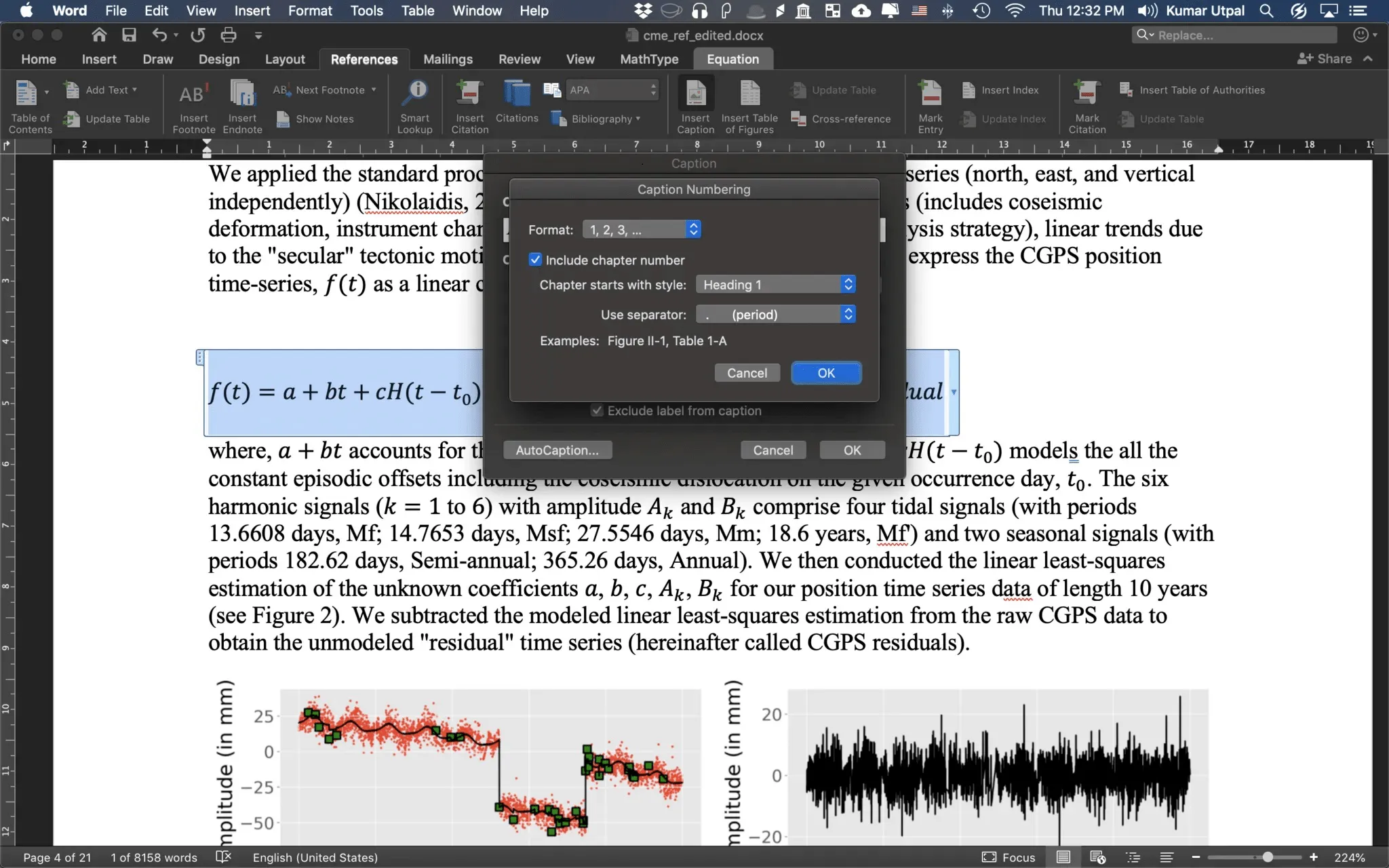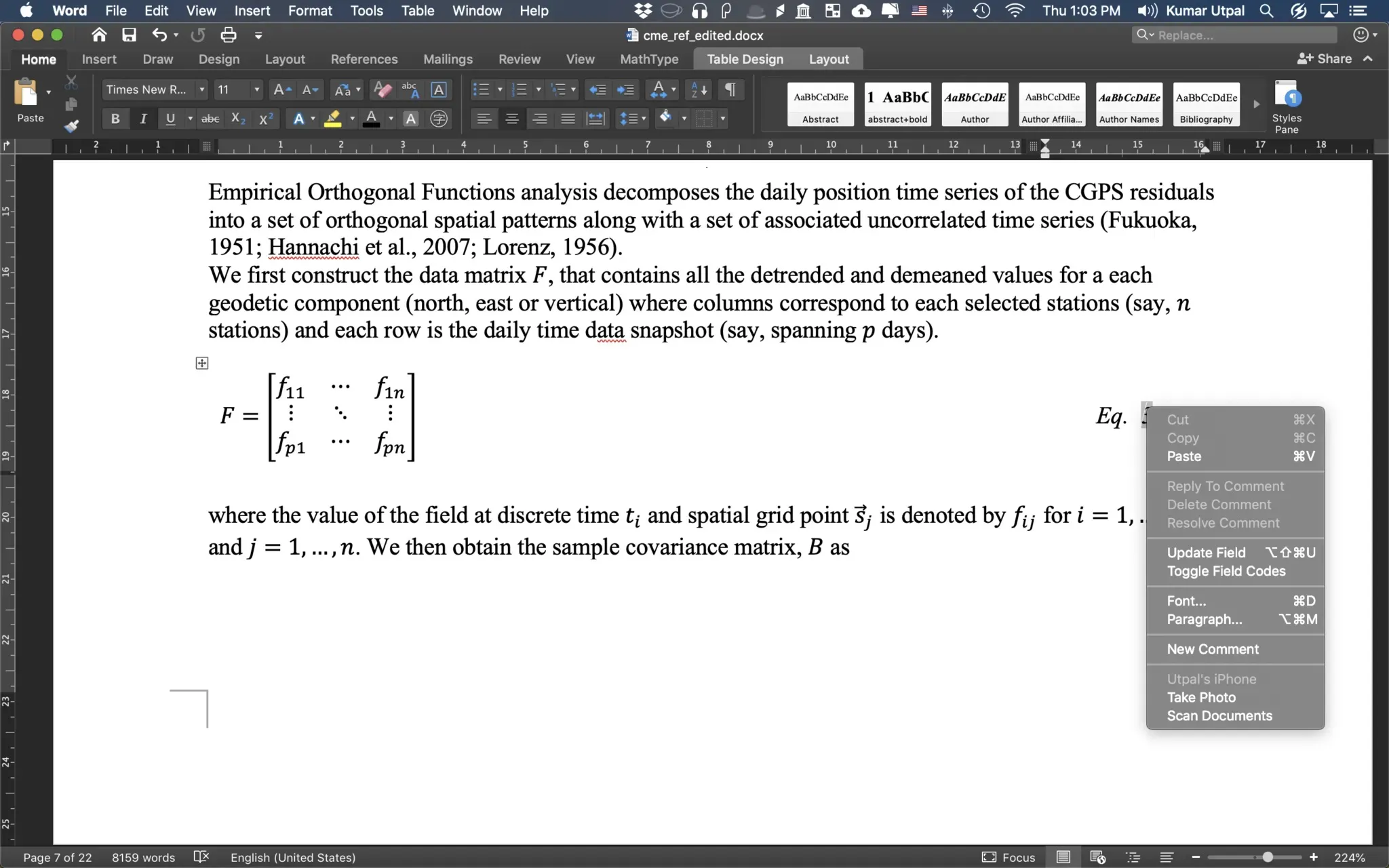How to insert equation numbering in MS Word

It is essential to insert equation numbers in your thesis and/or any scientific paper. In this post, I will show you some of the easiest ways to insert equations. Templates are available to use directly.

Introduction

It is essential to insert equation numbers if you are working on your thesis and/or any scientific paper consisting of a lot of equations. If your paper has many equations, then probably the best and the easiest way for you would be to write your manuscript in latex. Latex can do it smoothly and efficiently. But MS word offers several features like a spelling and grammar checker, easy writing without memorizing the codes for different tasks that have a definite advantage over the latex. The most important of all is the collaborative purpose. Almost all people are familiar with MS word, but only a fraction of our collaborators are familiar with the latex.

Similar posts

Writing scientific papers in ulysses

There are many new apps such as Ulysses, etc that rely on Markdown to provide all sorts of tools to easily write manuscript. Many writers love Ulysses more than Word or Latex, as they can finish their task much faster.

MS Word has been evolving fast. It is now quite responsive for longer documents (one of my biggest complaints with older versions of MS Word), and now it offers the insertion of equations in the latex syntax. They are adding more and more features with time. Probably add-ons are my next favorite feature.

Writing and formatting a scientific manuscript in Microsoft Word

If you are ready to use the Microsoft Word as your favourite tool for writing your awesome scientific thoughts and ideas into a manuscript, then I would like...

Download the word file for quick insertion of the format:Step-by-step demo

I have a manuscript where I want to insert several equations in order. Following is the step by step tutorial of how to insert auto-numbering to the equations. For the details on how to write a scientific manuscript with steps on how to properly include Figures, Tables, Citations, etc, visit my another post.

Let’s first start with one equation. The aim is to create a template that can be used to automatically generate the table and equation with equation number to the right.

1. We select the equation, and then go to the references tab
2. We click on the `Insert Caption` option and select the `label` as an equation. We can exclude the label from the caption if desired.
3. We can also edit the numbering format.Equation number format We can select to include the chapter number where the chapter starts with heading 1 numbering and use the separator as "period". Here, I chose to exclude the chapter number in the numbering.

4. Now, we insert the table with three columns and format the cell size according to our requirement.
5. Now, we cut and paste the equation and equation number in the second and third column respectively.
6. Now, we need to align everything. We do this by selecting the table and going to the layout tab and `align center`.

7. For the table, we don’t need a border, so remove it.
8. Now, we have an equation and its number. We can now write as many equations as we like by just copy and paste the format. We can right click and update the field to get the ordered numbering of equations.Update equation numbers We can also edit the equation label and use `Eq.` instead of just a number.

If you want to create high-resolution maps for your manuscript (two or three dimensional), you can copy the python script from here and modify the data and location coordinates to obtain beautiful map for your manuscript.

I have also created a list of templates for plotting two-dimensional XY plots using Python.

Template for easy insertion of equations

Create equation template in MS word... x
Create equation template in MS word for quick insertion of equations #Word #equations
• We can save the equation to the equation gallery for later use as a template. To do this:
• Highlight the equation table
• Select Insert → Equation → Save Selection to Equation Gallery

Create equation template for quick insertion

We can save the equation to the equation gallery for later use as a template. To do this:

• Highlight the equation table
• Select Insert → Equation → Save Selection to Equation Gallery

Tags:

Categories:

Created on:Disclaimer of liability

The information provided by the Earth Inversion is made available for educational purposes only.

Whilst we endeavor to keep the information up-to-date and correct. Earth Inversion makes no representations or warranties of any kind, express or implied about the completeness, accuracy, reliability, suitability or availability with respect to the website or the information, products, services or related graphics content on the website for any purpose.

UNDER NO CIRCUMSTANCE SHALL WE HAVE ANY LIABILITY TO YOU FOR ANY LOSS OR DAMAGE OF ANY KIND INCURRED AS A RESULT OF THE USE OF THE SITE OR RELIANCE ON ANY INFORMATION PROVIDED ON THE SITE. ANY RELIANCE YOU PLACED ON SUCH MATERIAL IS THEREFORE STRICTLY AT YOUR OWN RISK.